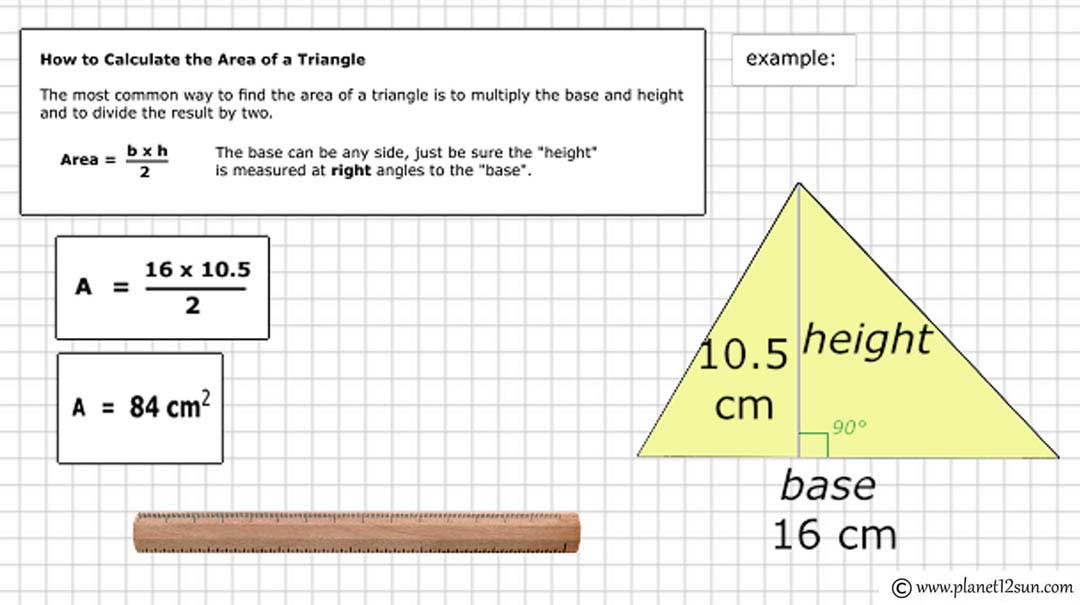## Geometric Shapes

Difference between 2D and 3D Shapes
Two-dimensional shapes have 2 dimensions, such as length and width, while three-dimensional shapes have an additional dimension, such as height. Any shape that only has a surface area is a 2-D shape while shapes with volume are 3-D shapes.

What is a Polygon?
A polygon is a 2-dimensional shape made of straight lines and the shape is "closed" (all the lines connect up). Triangles, rectangles, pentagon, hexagon etc. are all polygons.

### Common 2D ShapesCircle
The circle is a round shape with only one side and no corners.
All points on the circle are the same distance from the center.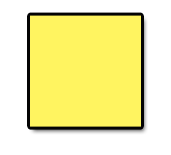Square
It has 4 right angles (90°) and 4 equal sides.
It is a special type of rectangle.Rectangle
It has 4 sides and 4 corners.
All the corners are right angles (90°).
Opposite sides are equal and parallel.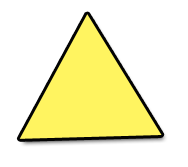Triangle
It has 3 sides and 3 angles.
The 3 angles always add to 180°.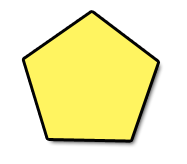Pentagon
A pentagon is a 5-sided polygon (a flat shape with straight sides).
It has 5 sides and 5 corners.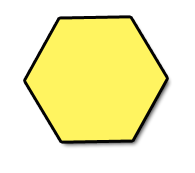Hexagon
A hexagon is a 6-sided polygon.
It has 6 sides and 6 corners.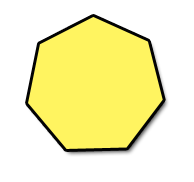Heptagon
A heptagon is a 7-sided polygon.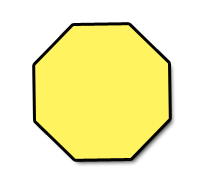Octagon
An octagon is an 8-sided polygon.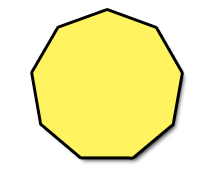Nonagon
A nonagon is a 9-sided polygon.Decagon
A decagon is a 10-sided polygon.

### Common 3D Shapes

What is a Prism?
A Prism is a three-dimensional shape, which consists of two identical polygons. Each one is called a base. These polygons do not lie in the same axis.
For each side of the polygon, there will be a parallelogram (4-sided shape with opposite sides parallel). All sides are flat- not curved.
examples: cube, rectangular prism, triangular prism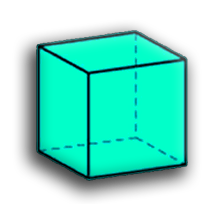Cube
It has 6 faces.
Each face has 4 edges (and is a square).
All sides are equal.
It has 12 edges.
It has 8 vertices (corner points).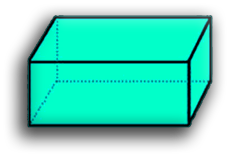Rectangular Prism (Cuboid)
A cuboid is a box-shaped object.
It has six flat faces and all angles are right angles.
All of its faces are rectangles.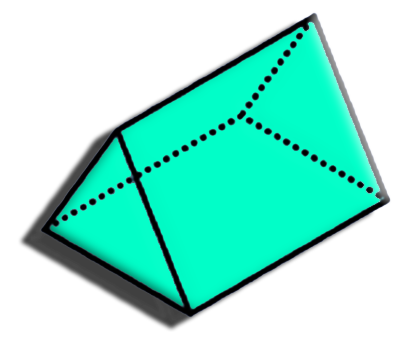Triangular Prism
A triangular prism is a prism composed of two triangular, parallel bases and three rectangular sides.Cone
It has a flat base.
It has one curved side.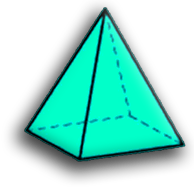Square Pyramid
It has 5 Faces.
The 4 side faces are triangles.
The base is a square.
It has 5 vertices (corner points).
It has 8 edges.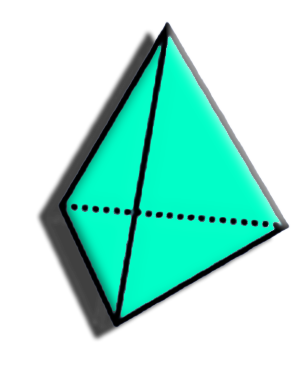Triangular Pyramid
It has three faces that are triangles and a base that is again a triangle.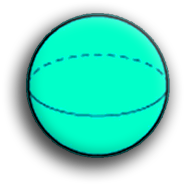Sphere
It is perfectly symmetrical.
All points on the surface are the same distance from the center.
It has no edges or vertices (corners).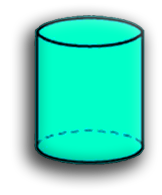Cylinder
It has a flat base and a flat top.
The base is the same as the top, and also in-between.
It has one curved side.

A quadrilateral is a four-sided polygon with four angles. Some quadrilaterals have special names because of their sides. Some have special names because of their angles.
Some types are also included in the definition of other types. For example a square, rhombus and rectangle are also parallelograms.

#### Examples: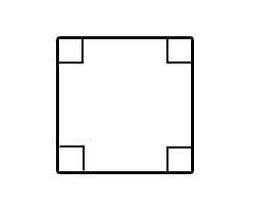Square
4 right angles and 4 equal sides.
It is also a rectangle and a parallelogram.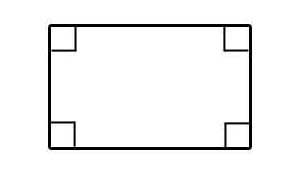Rectangle
4 right angles and opposite sides equal.
It is also a parallelogram.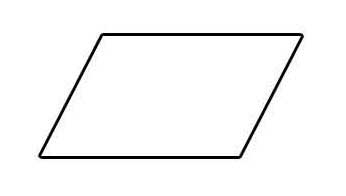Parallelogram
2 pairs of parallel sides and opposite sides equal.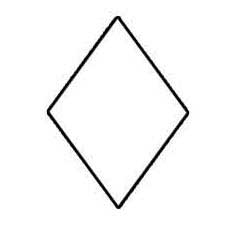Rhombus
2 pairs of parallel sides, all sides equal.
It is also a parallelogram.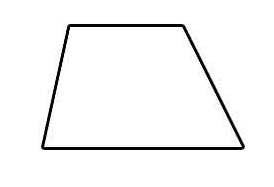Trapezoid
Only 2 sides are parallel.
It is a type of quadrilateral that is not a parallelogram.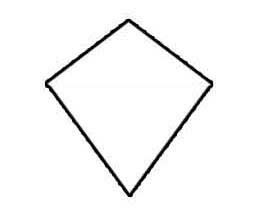Kite
2 pairs of adjacent sides are equal.

### Triangles

A triangle has three sides and three angles and is made of straight lines. The three angles always add to 180°. A triangle may be classified by how many of its sides are of equal length. Or, it may be classified by what kind of angles it has.

Types of Triangles by Length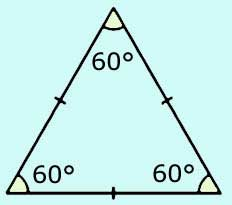Equilateral Triangle
Three equal sides.
Three equal angles, always 60°.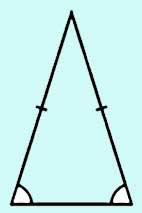Isosceles Triangle
Two equal sides.
Two equal angles.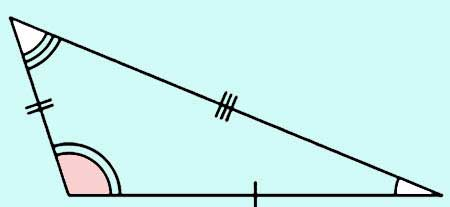Scalene Triangle
No equal sides.
No equal angles.

Types of Triangles by Angle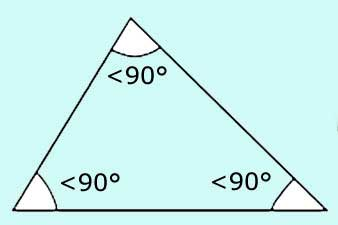Acute Triangle
All angles are less than 90°.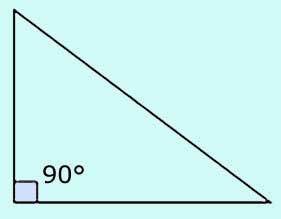Right Triangle
Has a right angle (90°).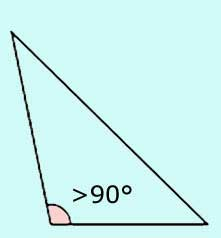Obtuse Triangle
Has an angle more than 90°.

Perimeter of a Triangle
The perimeter is the distance around the edge of the triangle. Just add up the three sides: p = a + b + c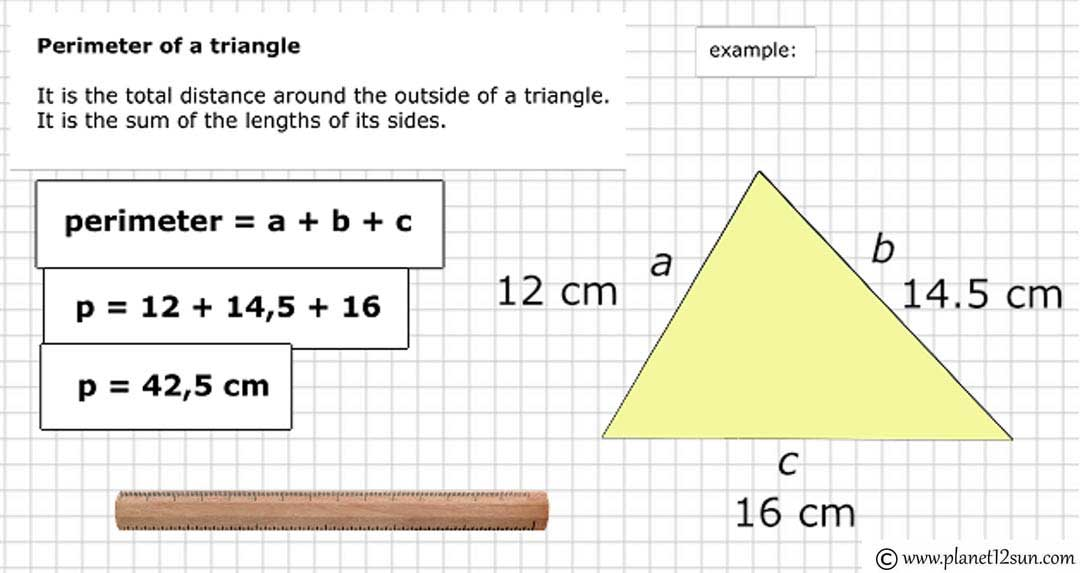Area of a Triangle
The most common way to find the area of a triangle is to multiply the base and height and to divide the result by two. A = 1/2 bh